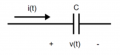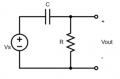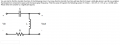# Sinusoidal Signals

#### kozza

Joined Oct 9, 2021
1
1)A voltage of v(t) = 4.4 cos (24 t – 43°) V is applied across a capacitor C = 6.7 mF as shown. What is the magnitude of the current phasor through the capacitor?2)
1. Given the circuit below, find the output voltage vout measured across the resistor. You are told that vs = 20 cos (10t + 40°), and you may assume that C = 39 μF and R = 1,128 Ω.Once you have found the output voltage, enter the magnitude of the output voltage (in volts) below, to 3 significant figures.
3)#### RBR1317

Joined Nov 13, 2010
674
Parts 1) & 2) seem to give more information than is needed to answer the question. Have you determined what you actually need to solve the problem?

#### dcbingaman

Joined Jun 30, 2021
497
How I would go about it:
1. Convert all voltage sources from polar form to complex form.
2. Express inductive reactance with jwL and capacitive reactance as -j/wL where w is angular frequency in radians per second
3. Use ohms law as usual just with complex numbers
4. For final answer convert complex form back to polar form where appropriate.
5. If answer requires hz convert radians per second to hz as needed.

Do you know how to perform those steps? Or is there some of those steps that you find hard to do?

#### dcbingaman

Joined Jun 30, 2021
497